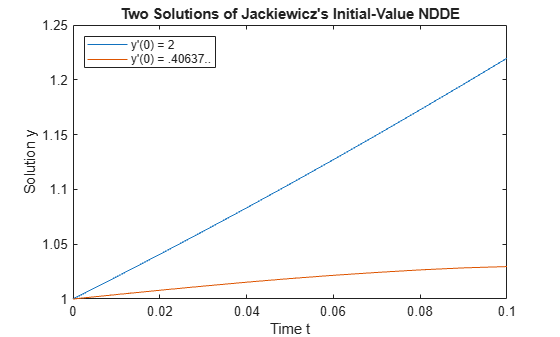# Initial Value DDE of Neutral Type

This example shows how to use `ddensd` to solve a system of initial value DDEs (delay differential equations) with time-dependent delays. The example was originally presented by Jackiewicz .

The equation is

`${\mathit{y}}^{\prime }\left(\mathit{t}\right)=2\text{\hspace{0.17em}}\mathrm{cos}\left(2\mathit{t}\right){\mathit{y}\left(\frac{\mathit{t}}{2}\right)}^{2\text{\hspace{0.17em}}\mathrm{cos}\left(\mathit{t}\right)}+\mathrm{log}\left({\mathit{y}}^{\prime }\left(\frac{\mathit{t}}{2}\right)\right)-\mathrm{log}\left(2\text{\hspace{0.17em}}\mathrm{cos}\left(\mathit{t}\right)\right)-\mathrm{sin}\left(\mathit{t}\right).$`

This equation is an initial value DDE because the time delays are zero at ${\mathit{t}}_{0}$. Therefore, a solution history is unnecessary to calculate a solution, only the initial values are needed:

`$\mathit{y}\left(0\right)=1,$`

`${\mathit{y}}^{\prime }\left(0\right)=\mathit{s}.$`

$\mathit{s}$ is the solution of $2+\mathrm{log}\left(\mathit{s}\right)-\mathrm{log}\left(2\right)=0$. The values of $\mathit{s}$ that satisfy this equation are ${\mathit{s}}_{1}=2$ and ${\mathit{s}}_{2}=0.4063757399599599$.

Since the time delays in the equations are present in a ${\mathit{y}}^{\prime }$ term, this equation is called a neutral DDE.

To solve this equation in MATLAB, you need to code the equation and delays before calling the delay differential equation solver `ddensd`, which is the solver for neutral equations. You either can include the required functions as local functions at the end of a file (as done here), or save them as separate files in a directory on the MATLAB path.

### Code Delays

First, write an anonymous function to define the delays in the equation. Since both $\mathit{y}$ and ${\mathit{y}}^{\prime }$ have delays of the form $\frac{\mathit{t}}{2}$, only one function definition is required. This delay function is later passed to the solver twice, once to indicate the delay for $\mathit{y}$ and once for ${\mathit{y}}^{\prime }$.

`delay = @(t,y) t/2; `

### Code Equation

Now, create a function to code the equation. This function should have the signature `yp = ddefun(t,y,ydel,ypdel)`, where:

• `t` is time (independent variable).

• `y` is the solution (dependent variable).

• `ydel` contains the delays for y.

• `ypdel` contains the delays for ${\mathit{y}}^{\prime }=\frac{\mathrm{dy}}{\mathrm{dt}}$.

These inputs are automatically passed to the function by the solver, but the variable names determine how you code the equation. In this case:

• `ydel`$\to \mathit{y}\left(\frac{\mathit{t}}{2}\right)$

• `ypdel`$\to {\mathit{y}}^{\prime }\left(\frac{\mathit{t}}{2}\right)$

```function yp = ddefun(t,y,ydel,ypdel) yp = 2*cos(2*t)*ydel^(2*cos(t)) + log(ypdel) - log(2*cos(t)) - sin(t); end ```

Note: All functions are included as local functions at the end of the example.

### Solve Equation

Finally, define the interval of integration $\left[{\mathit{t}}_{0}\text{\hspace{0.17em}\hspace{0.17em}}{\mathit{t}}_{\mathit{f}}\right]$ and the initial values, and then solve the DDE using the `ddensd` solver. Pass the initial values to the solver by specifying them in a cell array in the fourth input argument.

```tspan = [0 0.1]; y0 = 1; s1 = 2; sol1 = ddensd(@ddefun, delay, delay, {y0,s1}, tspan);```

Solve the equation a second time, this time using the alternate value of $\mathit{s}$ for the initial condition.

```s2 = 0.4063757399599599; sol2 = ddensd(@ddefun, delay, delay, {y0,s2}, tspan);```

### Plot Solution

The solution structures `sol1` and `sol2` have the fields `x` and `y` that contain the internal time steps taken by the solver and corresponding solutions at those times. However, you can use `deval` to evaluate the solution at the specific points.

Plot the two solutions to compare results.

```plot(sol1.x,sol1.y,sol2.x,sol2.y); legend('y''(0) = 2','y''(0) = .40637..','Location','NorthWest'); xlabel('Time t'); ylabel('Solution y'); title('Two Solutions of Jackiewicz''s Initial-Value NDDE');```### Local Functions

Listed here are the local helper functions that the DDE solver `ddensd` calls to calculate the solution. Alternatively, you can save these functions as their own files in a directory on the MATLAB path.

```function yp = ddefun(t,y,ydel,ypdel) yp = 2*cos(2*t)*ydel^(2*cos(t)) + log(ypdel) - log(2*cos(t)) - sin(t); end```

### References

 Jackiewicz, Z. “One step Methods of any Order for Neutral Functional Differential Equations.” SIAM Journal on Numerical Analysis. Vol. 21, Number 3. 1984. pp. 486–511.